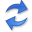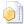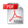MDL for Causal Inference on Discrete Data

Abstract. The algorithmic Markov condition states that the most likely causal direction between two random variables $$X$$ and $$Y$$ can be identified as the direction with the lowest Kolmogorov complexity. That is, if $$X$$ causes $$Y$$, $$K(P(X))+K(P(Y\mid X))$$ will be lower than $$K(P(Y))+K(P(X\mid Y))$$. This notion is very powerful as it can detect any causal dependency that can be explained by a physical process. However, due to the halting problem, it is also not computable. In this paper we propose a computable instantiation of this idea, based on stochastic complexity, that provably maintains the key aspects of the ideal.

That is, in this paper we propose to approximate Kolmogorov complexity via the Minimum Description Length (MDL) principle, using a score that is mini-max optimal with regard to the model class under consideration. This means that even in an adversarial setting, such as when the true distribution is not in our chosen model class, the score degrades gracefully, and we are still maximally able to detect dependencies between the marginal and the conditional distribution.

As a proof of concept, we propose CiSC, a linear-time algorithm for causal inference by stochastic complexity, for pairs of univariate discrete variables. Experiments show that CiSC is highly accurate on synthetic, benchmark, as well as real-world data, outperforming the state of the art by a margin, and scales extremely well with regard to sample and domain sizes.

## Implementationthe Python source code (February 2017) by Kailash Budhathoki.

## Related PublicationsBudhathoki, K Causal Inference on Discrete Data. Dissertation, Saarland University, 2020.Budhathoki, K & Vreeken, J Accurate Causal Inference on Discrete Data. In: Proceedings of the IEEE International Conference on Data Mining (ICDM'18), IEEE, 2018. (19.9% acceptance rate)Budhathoki, K & Vreeken, J MDL for Causal Inference on Discrete Data. In: Proceedings of the IEEE International Conference on Data Mining (ICDM'17), pp 751-756, IEEE, 2017. (19.9% acceptance rate)Budhathoki, K & Vreeken, J Causal Inference by Stochastic Complexity. Technical Report 1702.06776, arXiv, 2017.